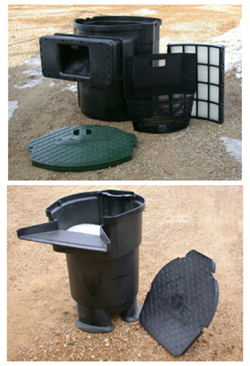Filtration

Biological vs. Mechanical Filtration

Filters are used to remove suspended particles from the water (mechanical) or they process the water biologically, removing nutrients and organic pollutants using bacteria.  A mechanical filter is attached to the intake of a pump and is submerged in the pond.  It may also be a canister filter that operates under pressure. These are typically positioned outside of the pond where the pump pushes water into the filter. The filtration media is fine-pore polyester that traps the particle until it is removed and rinsed away.  A biological filter uses the same material but is located outside of the pond on the outlet side of the pump and is not operated under pressure.  The water flows through the material, which contains a colony of beneficial bacteria that cleans the water of pollutants.  Ideally a biological filter would be pre-filtered with a mechanical filter to keep the media as clean as possible to maintain peak efficiency. A skimmer does this job very well.

Figuring the Pond Volume

For a rectangle or square, measure the pond length, width and depth in feet.  Multiply the three numbers to obtain the cubic volume of the water.  Multiply this number by 7.5 to get the total number of gallons of water.  If the surface of the pond is irregular, try to estimate the actual square footage as close as possible before multiplying with the depth.  The depth should be an average depth, not the deepest point.

The formula for calculating the volume of your pond is:

L = Average Length

W = Average Width

D = Average Depth

x = multiply

V = Volume (in Gallons of Water)

L x W x D x 7.5 = V

QUICK FORMULA FOR POND SHAPES, SURFACE AREA:

• Square or rectangle is L X W = square feet
• Oval is 3.14 X radius (horizontal) X radius (vertical) = square feet
• Triangle is base X height X 1/2 = square feet

For an irregular pond, take an average of several measurements from length and for the width. Multiply the average measurements together to get square feet of an irregular shape.An alternative to measuring pond volume is to use a water gauge for the garden hose that can be purchased at a hardware store. Another possibility is to record the amount of time it takes to fill a five gallon bucket, then time the filling of the entire pond and divide the total number of minutes by the time it took to fill the bucket by 5.Hours

Tues - Sat:  9 AM - 5 PM

Closed Sundays and Mondays

Closed July 4th

Closed July 24th to August 1st